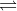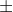In: Chemistry

# Calculate the concentration of all species present (H2SO4, HSO4^-, H^+, SO4^-2) in a 0.285 M H2SO4...

Calculate the concentration of all species present (H2SO4, HSO4^-, H^+, SO4^-2) in a 0.285 M H2SO4 solution. The diprotic acid equilibrium is :

H2SO4 <--> H^+ + HSO4^- Ka = very large

HSO4^- <---> H^+ + SO4^-2 Ka = 1.2 x 10^-2

Please explain why and when we use +x and -x. I am very confused on this.

## Solutions

##### Expert Solution

H2SO4H+ + HSO4-    Ka1 = very large (about 1 x 103)

HSO4-H+ + SO4-2    Ka2 = 1.2 x 10-2

Given, concentration of H2SO4 = 0.285 M

For the first equibrium,

H2SO4H+ + HSO4-

let x parts of H2SO4 get dissociated

So, from Initial change and equilibrium table or ICE Table

 Particulars H2SO4 H+ HSO4- Initial 0.285 0 0 change -x +x +x equilibrium 0.285-x +x +x

-x implies that x part of the reactant gets dissociated or lost from it. Here H2SO4 loses x part to give x parts of H+   and HSO4- each

+x implies that x part of the products formed . Here x parts of both H+   and HSO4- are formed from x part of H2SO4. let's say 1 unit gets dissociated from H2SO4. So, 1 unit each of both H+   and HSO4- are formed.

+x is used when reactant gets dissociated and -x is used products are formed

now, since H2SO4 is a strong acid, hence its dissociation is complete,

i.e. 0.285-x = 0 or x = 0.285 M

For second reaction,

HSO4-H+ + SO4-2

let y parts of HSO4- gets dissociated now

From ICE table,

 Particulars HSO4- H+ SO4-2 Initial x x 0 Change -y +y +y Equilibrium x-y x+y +y

Ka2 = [H+][SO4-2 ]/[HSO4-]

1.2 x 10-2 = (x+y)(y)/(x-y)

= (0.285+y) y / (0.285-y)

or, y2 + 0.297 y-0.00342 = 0

y= [-0.297{0.2972 - (4*1*(-0.00342))}1/2]/2

= (-0.2970.319) / 2

or, y = -0.616 or 0.022

since, concentration cannot be negative, y= 0.022 M

Hence, at equilibrium,

[H2SO4] = 0 M,

[H+] = x+y = 0.285 + 0.022 = 0.307 M

[HSO4- ] = x-y = 0.285 -0.022 = 0.263 M

[SO4-2] = y = 0.022 M

## Related Solutions

##### Calculate the pH and the concentrations of all species present in 0.18 M H 2 SO...
Calculate the pH and the concentrations of all species present in 0.18 M H 2 SO 3 . ( K a1 = 1.5× 10 −2 , K a2 = 6.3× 10 −8 ) Express your answer to three significant figures and include the appropriate units
##### Use two concentration tables to calculate the concentrations of all species present in a 0.0774 M...
Use two concentration tables to calculate the concentrations of all species present in a 0.0774 M solution of KBrO. The Ka for HBrO is 2.8E-9. What is the concentration of K+? What is the concentration of BrO-?
##### Calculate the concentration (in M) of each species present in a 0.085 M solution of H2GeO3....
Calculate the concentration (in M) of each species present in a 0.085 M solution of H2GeO3. (See the Acid and Base Dissociation Constants table. Assume Kw = 1.01 ✕ 10−14.) [H2GeO3]______ M [HGeO3− ]______ M [GeO32− ]______ M M [H3O+ ]______ M M [OH − ]_______ M
##### Calculate the pH of a 9.06×10-3 M solution of H2SO4. (Ka = 0.0120 for HSO4-)
Calculate the pH of a 9.06×10-3 M solution of H2SO4. (Ka = 0.0120 for HSO4-)
##### Part A Calculate the pH and the concentrations of all species present in 0.16 M H2SO3....
Part A Calculate the pH and the concentrations of all species present in 0.16 M H2SO3. (Ka1 = 1.5×10−2, Ka2 = 6.3×10−8) Express your answer to three significant figures and include the appropriate units. pH = SubmitMy AnswersGive Up Incorrect; Try Again; 4 attempts remaining Part B Calculate the concentration of H2SO3 in solution. Express your answer to two significant figures and include the appropriate units. SubmitMy AnswersGive Up Incorrect; Try Again; 5 attempts remaining Part C Calculate the concentration...
##### Calculate the concentration of all species in a 0.490 M solution of H2SO3. The acid ionization...
Calculate the concentration of all species in a 0.490 M solution of H2SO3. The acid ionization constants for the acid are Ka1=1.6×10−2 and Ka2=6.4×10−8. [H2SO3], [HSO−3], [SO2−3], [H3O+], [OH−]
##### calculate the concentration of all species in a 0.175 M solution of H2CO3. H2CO3 HCO3- CO3...
calculate the concentration of all species in a 0.175 M solution of H2CO3. H2CO3 HCO3- CO3 ^2- H3O- OH-
##### Calculate the concentration of all species in a 0.130M solution of H2CO3. [H2CO3], [HCO3^-] , [CO3^2-],...
Calculate the concentration of all species in a 0.130M solution of H2CO3. [H2CO3], [HCO3^-] , [CO3^2-], [H3O^+], [OH^-] Please show work
##### Calculate the concentration of all species in a 0.180 M solution of H2CO3. [H2CO3], [HCO−3], [CO2−3],...
Calculate the concentration of all species in a 0.180 M solution of H2CO3. [H2CO3], [HCO−3], [CO2−3], [H3O+], [OH−]
##### calculate the concentrations of all species present at equilibrium for a .200M solution of sodium arsenate,...
calculate the concentrations of all species present at equilibrium for a .200M solution of sodium arsenate, Na3AsO4. ( Don't forget the hydrolysis reactions of the arsenate ion)